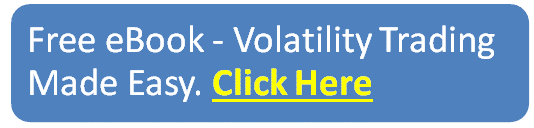blog

# Ultimate Guide To Call Ratio Backspreads

#### Options Trading 101 - The Ultimate Beginners Guide To Options

As Seen On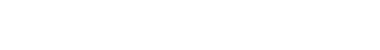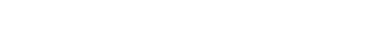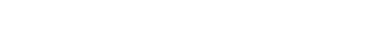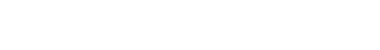by Gavin in Blog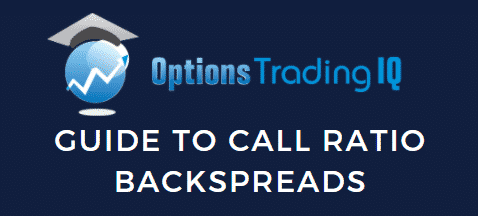## Contents

A call ratio backspread is an option trade that involves buying call options and selling a different number of call options on the same instrument and the same expiration date.

The name of the trade comes from the ratio between the number of long and short positions.

There are two types of call ratio spreads, the first one is the call front ratio spread or simply call ratio spread, and the second one is the call ratio backspread.

The most common ratio in call ratio spreads is two-to-one, where there are twice as many short calls as long ones

The strategy is profitable when the stock rallies above the long calls strike price or the implied volatility increases.

The trade is a combination of a bear call spread and a long call out-of-the-money.

A typical call ratio spread is created selling one at-the-money call option and buying two call options at a higher strike price.

If the trade is placed for a credit, there is no downside risk in the trade.

There is some risk if the stock moves closer to the strike price of the long call options or if implied volatility decreases.

Some investors prefer this strategy over buying long calls because the trade can be initiated for a credit.

Here is an example of a SPY call ratio backspread.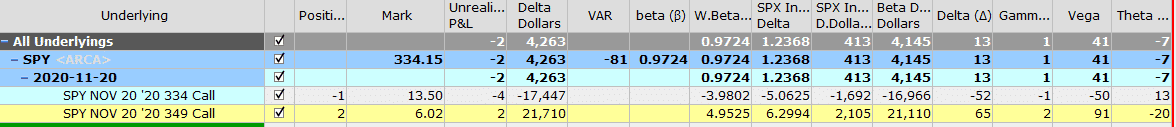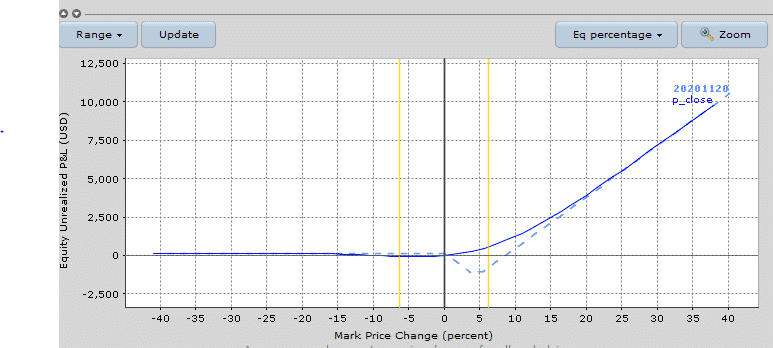## Maximum Loss

The maximum loss happens when at expiry the stock is at the strike price of the long calls.

The maximum loss is equal to the intrinsic value of the short call plus or minus the net debit or credit received when the trade was initiated.

In the SPY case, there is no loss on the downside because the trade was created for a credit.

If the trade was created for credit, the maximum loss is:

Maximum loss = Strike price long calls – strike price short call – Net credit received

Our SPY credit ratio backspread was initiated for a credit of \$146, the short SPY 334 call, and long SPY 349 calls are \$15 apart. The maximum loss of the trade would be:

Max loss = \$1500 – \$146 = \$1354

If the trade was initiated for a debit, the maximum loss is:

Maximum loss = Strike price long calls – strike price short call + Net premium paid

## Maximum Gain

The maximum gain is unlimited. It occurs when the stock makes a strong move above the strike price of the long calls. In this situation, the short call would expire at the money as well as the and long calls.

## Breakeven Price

If the call ratio backspread is established for a credit, there are two breakeven prices which are:

Upper breakeven price = strike price of the long calls + difference between the strikes – net credit received

Lower breakeven price = strike price of short long call + Net credit received

In our SPY example, the upper breakeven price is:

349 + 15 – 1.46 = \$362.06

In our SPY trade, the lower breakeven price is

334 + 1.46 = \$335.46

If the call ratio backspread is established for a debit, there is only one breakeven point:

Upper breakeven price = strike price of the long calls+ difference between the strikes – net debit paid

## Payoff Diagram

Call ratio backspreads have an inverted tent-shaped payoff diagram.

This kind of shape results in high gamma near expiry if the trade is within the inverted tent.

It the trade is created for a credit, call ratio backspreads have no risk on the downside.

The risk appears when the stock price stays between the two breakeven prices, that is, close to the long call strike.

For this reason, you initiate the trade when you are strong bully on the stock.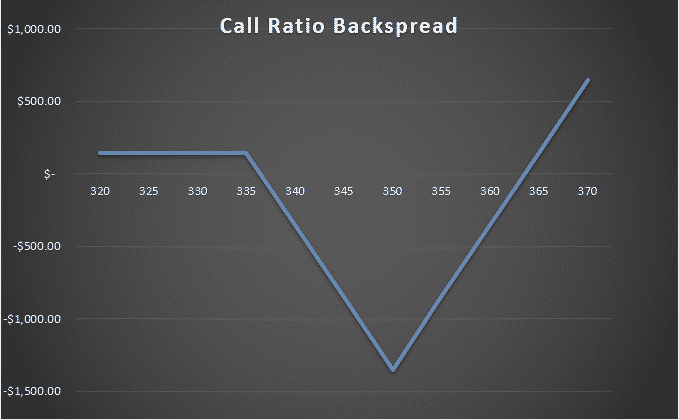## Risk of Early Assignment

There is always a risk of early assignment when the price of the underlying is above the strike price of the short call.

Usually, an early assignment only occurs on call options when there is an upcoming dividend payment.

Traders will exercise the call to take ownership of the share before the ex-date and receive the dividend.

For this reason, it is important to watch out for ex-dividend dates.

Otherwise, make sure to close the trade if the stock price is between the breakeven prices and the trade is close to the expiration date.

## How Volatility Impacts Call Ratio Backspreads

Call ratio backspreads are long vega trades, so generally speaking, they benefit from rising volatility after the trade has been placed.

Our SPY example starts with a vega of 41.

This means that for every 1% increase in implied volatility, the trade should gain \$41.

The opposite is true if implied volatility drops 1% – the position would lose \$41.

## How Theta Impacts Call Ratio Spreads

Call ratio backspreads are negative theta trades, they lose money as time passes, with all else being equal.

This is because the long calls suffer faster time decay than the short call.

In our SPY example, the trade has theta of negative 7.

This means that all else being equal, the trade will lose \$7 per day due to time decay.

Notice that the negative time decay on the long calls is higher than the time decay being suffered on the short call.

One small caveat is that the trade will actually show positive theta if the stock is below the lower breakeven price and the trade was initiated for a credit.

## Other Greeks

### DELTA

Delta on a call ratio backspread is usually positive when the trade is initiated.

As the trade progresses, the delta will change and it could potentially become negative.

Our SPY trade has a initial delta of 13. This means that all else being equal, for every \$1 increase in the price of SPY, the trade will make \$13.

### GAMMA

Call ratio backspreads are positive gamma trade, they benefit from changes in the delta of the position.

In other words, you want the stock to move, hopefully above the upper breakeven point.

In our SPY example, the initial call ratio spread had gamma of 1.

## Risks

The main risk with the trade is that the stock does not move high enough and it trades close to the strike price of the long calls.

### ASSIGNMENT RISK

Assignment risk is highest if the stock is trading ex-dividend because the short call is in the money.

One way to avoid assignment risk is to trade stocks that do not pay dividends or trade indexes that are European style and cannot be exercised early.

To reduce assignment risk consider closing your trade the stock is trading between the breakeven points close to the expiration date. If the position is profitable close to expiration, simply close the trade.

### EXPIRATION RISK

Leading into expiration, if the stock is trading close to the long strike call, the trader has expiration risk.

As the time of expiration approaches, the gamma of the at-the-money calls increases while the gamma of the in the money call decreases. Professional traders try to avoid gamma risk and they usually close their positions before the expiration week.

### VOLATILITY RISK

A call ratio spread is a positive vega strategy, this means that the position benefits from a rise in implied volatility. The combined vega of the long calls will generally be much greater than the short call. The volatility of the position changes with the duration of the trade.

## Long Call vs Call Ratio Backspread

There some important differences between long calls and call ratio backspreads.

Call ratio backspreads can be seen as a bear call spread and a long call.

The net credit obtained from selling the bear call in the call ratio backspread is usually bigger than the price paid for the extra long call.

This usually permits the trader to create the trade for a credit.

Theoretically, both trades have unlimited maximum gain on the upside but call ratio backspreads have a higher breakeven point than the long call trade.

Looking at our SPY call ratio backspread we have that the upper breakeven point was \$362.06 against a breakeven of

349 + 6.02 = \$355.02, consisting of the premium we paid for buying the SPY 349 call plus the long call strike price.

The breakeven point of buying the 334 call would be 334 + 13.50 = 347.50.

Call ratio backspreads have a higher maximum loss than long calls.

Our SPY call ratio backspread had a maximum loss of \$1354 against \$602 we would suffer from buying the 349 call.

If the trader decides to buy the 334 SPY call, it would cost \$1350.

One of the biggest advantages of call ratio backspreads over long call is that the trade could be profitable even if the stock drops below the strike price of the call.

Long call positions are usually expensive, many traders find call ratio backspreads a more convenient way take a bullish exposure on a stock.

As with all trading strategies, it is important to plan how you are going to manage the trade in any scenario.

What will you do if the stock does not move? Where will you take profits? Where and how will you adjust? When will you get stopped out?

Let us discuss some of the basics of how to manage call ratio backspreads.

### PROFIT TARGET

It is important to have a profit target. That might be 20% of capital at risk or you may plan on holding to expiration provided the stock stays above the long strike calls. For call ratio backspreads, 20 percent is a good target.

For call ratio backspreads, 20% of capital at risk should be calculated base on the maximum loss that the trade can suffer.

In our SPY trade, we received a net credit of \$146 with a maximum risk of \$1354, the trade would need to make

\$270 if you expect a 20% profit.

### STOP LOSS

Having a stop loss is also important, perhaps more so than the profit target.

With a call ratio backspread, you can set a stop loss based on a percentage of the capital at risk. In this case, it might be closing the trade if the loss reaches 15-20%, this will happen if the stock does not move.

With a call ratio backspread, the loss could be set based on the maximum possible loss of the trade. It is advisable to close the trade if it reaches a 15-20% loss. In our SPY trade, a 15% would mean a loss of \$200.

## Example

Let us look at a call ratio backspread trade in Microsoft. On August 11th 2020, Microsoft (MSFT) was trading at a price of 208.25.

Based on the fact that stock MSFT suffered a big drop in price, we created a call ratio backspread selling the ATM September 18th 210 call and buying two 225 calls with the same expiry.

The net premium for creating the trade was \$187.50 and the trade had a delta of 1.48 and a negative theta of 6.76.

The breakeven points of the trade are 225 + 15 – 1.88 = \$238.12. and 210 + 1.88 = \$211.88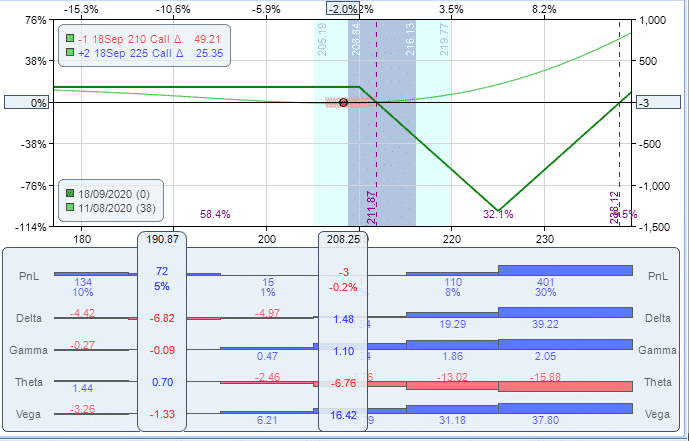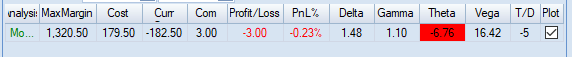As time goes, theta erodes the premium received when we started the trade, the price of the short calls starts losing value faster than the short call.

After two weeks, the trade has lost \$91 mainly because of the effect of theta and a drop in vega.

Delta has a value of negative 8.69.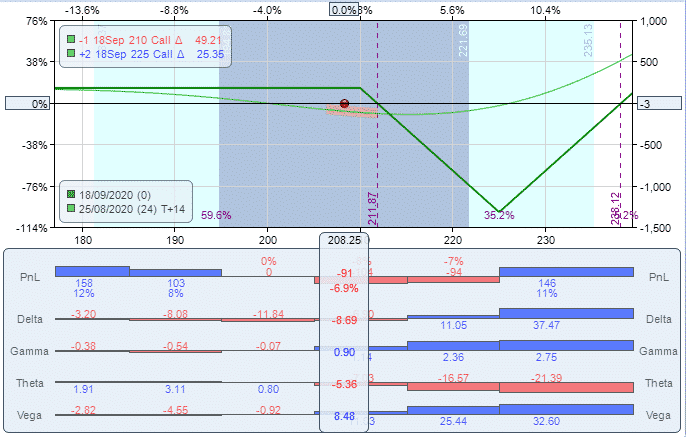The trade continues losing money but the stock is trading outside the breakeven points of 211. 88 and 238.12 during the expiration week.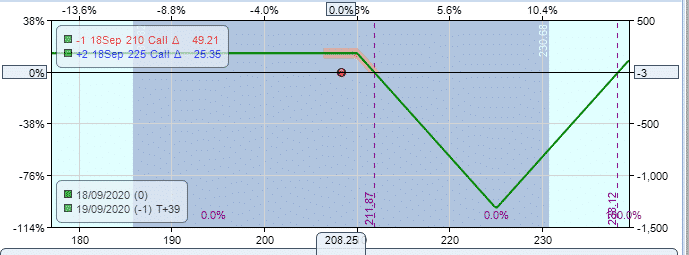At expiration the MSFT traded at \$203, a price below 210 which was the short call of the spread, we collected the total premium of \$180.

The trade was profitable although the stock did not rally.

## Summary

Call ratio backspreads are trading strategies that you can consider when you expect the stock will rally and you want protection on the downside.

For this reason, they should only be placed on stocks the trader thinks will move higher.

A good time to enter this type of trade is at the end of a pullback move when the trader expects a big upside move.

One nice feature of the trade is that there is very little or no risk on the downside and sometimes, even the ability to generate a small amount of premium if the stock drops.